# DAV Class 8 Maths Chapter 2 Worksheet 2 Solutions

The DAV Class 8 Maths Book Solutions and DAV Class 8 Maths Chapter 2 Worksheet 2 Solutions of Cubes and Cube Roots offer comprehensive answers to textbook questions.

## DAV Class 8 Maths Ch 2 WS 2 Solutions

Question 1.
Find the cube root of the following by prime factorisation:
(i) 5832
(ii) 1728
(iii) 216000
(iv) 21952
Solution:
(i) 5832

= $$\sqrt{5832}$$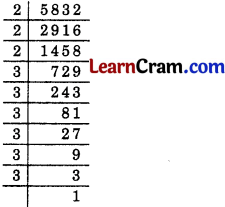= $$\sqrt{2 \times 2 \times 2 \times 3 \times 3 \times 3 \times 3 \times 3 \times 3}$$
= $$\sqrt{2^3 \times 3^3 \times 3^3}$$
= 2 × 3 × 3 = 18
Hence $$\sqrt{5832}$$ = 18

(ii) 1728
= $$\sqrt{1728}$$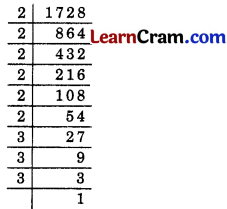= $$\sqrt{2 \times 2 \times 2 \times 2 \times 2 \times 2 \times 3 \times 3 \times 3}$$
= $$\sqrt{2^3 \times 2^3 \times 3^3}$$
= 2 × 2 × 3 = 12(iii) 216000
= $$\sqrt{216000}$$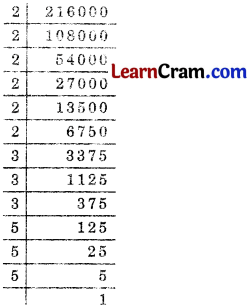=$$\sqrt{2 \times 2 \times 2 \times 2 \times 2 \times 2 \times 3 \times 3 \times 3 \times 5 \times 5 \times 5}$$
= $$\sqrt{2^3 \times 2^3 \times 3^3 \times 5^3}$$
= 2 × 2 × 3 × 5 = 60

Hence $$\sqrt{216000}$$ = 60

(iv) 21952
= $$\sqrt{21952}$$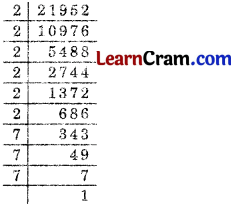= $$\sqrt{2 \times 2 \times 2 \times 2 \times 2 \times 2 \times 7 \times 7 \times 7}$$
= $$\sqrt{2^3 \times 2^3 \times 7^3}$$
= 2 × 2 × 7 = 28Question 2.
Find the cube root of the following integers:
(i) – 1728
(ii) – 2744000
(iii) – 474552
(iv) – 5832
Solution:
(i) – 1728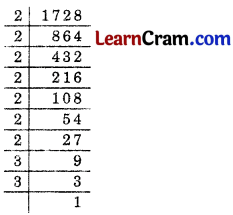= $$\sqrt{-1728}=\sqrt{-1} \times \sqrt{1728}$$
= – $$\sqrt{2 \times 2 \times 2 \times 2 \times 2 \times 2 \times 3 \times 3 \times 3}$$
= – $$\sqrt{2^3 \times 2^3 \times 3^3}$$
= – 2 × 2 × 3 = – 12

(ii) – 2744000
= $$\sqrt{-2744000}$$= $$\sqrt{-1} \times \sqrt{2744000}$$
= – $$\sqrt{2 \times 2 \times 2 \times 2 \times 2 \times 2 \times 5 \times 5 \times 5 \times 7 \times 7 \times 7}$$
= – $$\sqrt{2^3 \times 2^3 \times 5^3 \times 7^3}$$
= – 2 × 2 × 5 × 7 = 140
Hence $$\sqrt{-2744000}$$ = – 140(iii) – 474552
= $$\sqrt{-474552}$$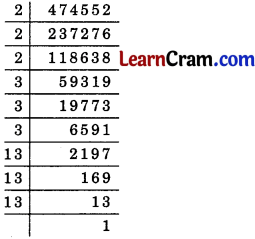= $$\sqrt{-1} \times \sqrt{474552}$$
= – $$\sqrt{2 \times 2 \times 2 \times 3 \times 3 \times 3 \times 13 \times 13 \times 13}$$
= – $$\sqrt{2^3 \times 3^3 \times 13^3}$$
= – 2 × 3 × 13 = – 78
Hence $$\sqrt{-474552}$$ = – 78

(iv) – 5832
= $$\sqrt{-5832}$$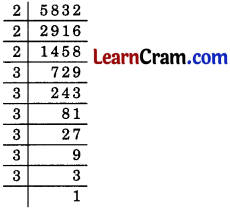= $$\sqrt{-1} \times \sqrt{5832}$$
= – $$\sqrt{2 \times 2 \times 2 \times 3 \times 3 \times 3 \times 3 \times 3 \times 3}$$
= – $$\sqrt{2^3 \times 3^3 \times 3^3}$$
= – 2 × 3 × 3 = – 18
Hence $$\sqrt{-5832}$$ = – 18Question 3.
Evaluate:
(i) $$\sqrt{8 \times 125}$$
(ii) $$\sqrt{3375 \times(-729)}$$
(iii) $$\sqrt{4^3 \times 5^3}$$
Solution:
(i) $$\sqrt{8 \times 125}$$(ii) $$\sqrt{3375 \times(-729)}$$= – 3 × 5 × 3 × 3 = – 135
Hence $$\sqrt{3375 \times(-729)}$$ = – 135.

(iii) $$\sqrt{4^3 \times 5^3}$$
= 4 × 5 = 20
Hence $$\sqrt{4^3 \times 5^3}$$ = 20Question 4.
Find the cube root of the following rational numbers:
(i) $$\frac{4913}{3375}$$
(ii) $$\frac{-512}{343}$$
(iii) $$\frac{-686}{-2662}$$
Solution:
(i) $$\frac{4913}{3375}$$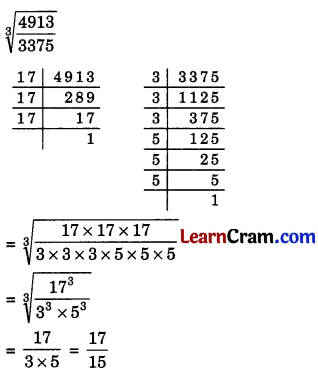Hence $$\sqrt{\frac{4913}{3375}}=\frac{17}{15}$$.

(ii) $$\frac{-512}{343}$$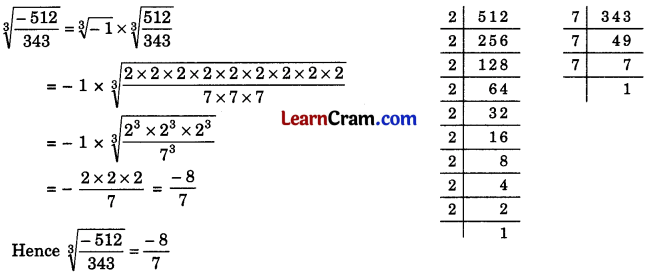(iii) $$\frac{-686}{-2662}$$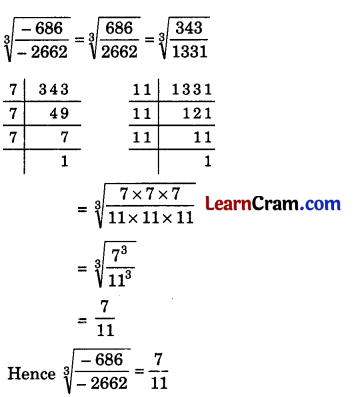Question 5.
By which smallest number must 5400 be multiplied to make it a perfect cube?
Solution: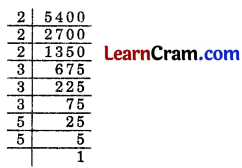5400 = 2 × 2 × 2 × 3 × 3 × 3 × 5 × 5
= 23 × 33 × 52
∴ 5 is the smallest required number.

Question 6.
Find the smallest number by which 16384 be divided sothat the quotient may be a perfect cube. 2 4096 2 64
Solution:16384 = 2 × 2 × 2 × 2 × 2 × 2 × 2 × 2 × 2 × 2 × 2 × 2 × 2
= 23 × 23 × 23 × 23 × 23
∴ 22 = 4 is the smallest required number.Question 7.
Find the cube root of the following numbers through estimation:
(i) 10648
(ii) 15625
(iii) 110592
(iv) 91125
Solution:
(i) 10648
= $$\sqrt{10648}$$

Step I. Let us take 3-digit number from the right of the given number i.e. 648 and consider it as I group.
II      I
10 648

Step II. Unit place in 648 is 8 which is cube of 2. Therefore, the unit place of the required number is 2.

Step III. Now take the second group i.e. 10
23 < 10 < 33
The smallest number between 2 and 3 is 2 which is taken as tens place of the required cube root.
Hence 22 is the required cube root of 10648.

(ii) 156225
= $$\sqrt{15625}$$

Step I. Let us take 3-digit number from the right of the given number i.e. 625 and consider it as I group and 15 as second group.
II      I
15 625

Step II. Unit place in 625 is 5
Now 53 = 125
So it also has 5 in its unit place

Step III. Now take 15
8 < 15 < 27
23 < 15 < 33
The smallest number between 2 and 3 is 2.
∴ 2 is in the tens place of the required number.
Hence 25 is the required cube root.(iii) 110592
= $$\sqrt{110592}$$

Step I. Let us take 3-digit number from the right of the given number i.e. 592 and consider it as I group and 110 in II group.
II       I
110 592

Step II. Unit place in 592 is 2
Now 23 = 8
So unit place of the required number is 8.

Step III. Now take the second group 110
64 < 110 < 125
43 < 110 < 53
The smallest number between 4 and 5 is 4
∴ 4 is in the second group of the required cube root.
∴ 48 is the required cube root of the given number.

(iv) 91125
= $$\sqrt{91125}$$

Step I. Let us take 3-digit number from the right of the given number i.e. 125 and consider it as I group and 91 in II group
II     I
91 125

Step II. Unit place in 125 is 5
Now 53 = 125 so it also has 5 in its unit place.
∴ 5 is in the unit place of the given cube root.

Step III. Now take 91 from II group
64 < 91 < 125
43 < 91 < 53
The smallest number between 4 and 5 is 4.
Therefore, 4 is in the ten’s place of the cube root of the given number.
Hence the required cube root = 45.### DAV Class 8 Maths Chapter 2 Value Based Questions

Question 1.
Students of a school collected provisions like rice, pulses, etc., for the flood affected people of Madhya Pradesh. These provisions were packed in six cubical cartons each of side measuring 65 cm.
(i) Find the volume of cartons packed.
(ii) What values do you learn from the students?
Solution:
(i) Side of one cubical carton = 65 cm
Volume of one cubical carton = (65 cm)3
= 65 cm × 65 cm × 65 cm = 274625 cm3
∴ Volume of 6 such cartons = 274625 × 6 = 1647750 cm3.

(ii) We can learn many values, like caring, helpful, sympathy, cooperation and responsibility from the students.Question 2.
A school decided to award prizes to students for three values-discipline, cleanliness of environment and regularity in attendance. The number of students getting prizes in the three categories are in the ratio 1 : 2 : 3. If product of ratios is 162, then
(i) Find the number of students getting prizes for each value.
(ii) Name any other two values that you can inculcate.
Solution:
(i) Let the number of students for each category be x, 2x and 3x such that
x × 2x × 3x = 162
⇒ 6x3 = 162
⇒ x3 = $$\frac{162}{6}$$ = 27
∴ x = $$\sqrt{3 \times 3 \times 3}$$ = 3
Number of students getting prizes for discipline = 3.
Number of students getting prizes for cleanliness = 2 × 27 = 54.
Number of students getting prizes for regularity = 3 × 27 = 81.

(ii) Others two values that we can inculcate are: (a) awareness (b) tolerance.